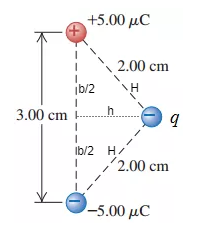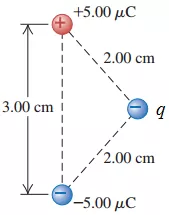# Problem: A) Find the magnitude of the net force that the q=−13.0μC charge exerts on the dipole.Express your answer in newtons to three significant figures.B) Find the direction of the net force that the q=−13.0μC charge exerts on the dipole.a) The force is in the direction from the + 5.00 μC to the − 5.00 μC charge.b) The force is in the direction from the + 5.00 μC to the − 13.0 μC charge.c) The force is in the direction from the − 13.0 μC to the − 5.00 μC charge.d) The force is in the direction from the − 5.00 μC to the + 5.00 μC charge.C) For an axis perpendicular to the line connecting the two charges of the dipole at its midpoint and perpendicular to the plane of the screen, find the magnitude of the torque exerted on the dipole by the − 13.0 μC charge.Express your answer in newton meters to three significant figures.

###### FREE Expert Solution

Coulomb's law:

$\overline{){\mathbf{F}}{\mathbf{=}}\frac{\mathbf{k}{\mathbf{q}}_{\mathbf{1}}{\mathbf{q}}_{\mathbf{2}}}{{\mathbf{r}}^{\mathbf{2}}}}$, where F is the force the charge exerts, k is Coulomb constant, and r is the separation between charges q1 and q2.

The direction of a vector is given by:

$\overline{){\mathbf{tan}}{\mathbit{\theta }}{\mathbf{=}}\frac{{\mathbit{A}}_{\mathbit{y}}}{{\mathbit{A}}_{\mathbit{x}}}}$

A.

Let's label the parts of the figure as shown:96% (78 ratings)###### Problem DetailsA) Find the magnitude of the net force that the q=−13.0μC charge exerts on the dipole.

Express your answer in newtons to three significant figures.

B) Find the direction of the net force that the q=−13.0μC charge exerts on the dipole.

a) The force is in the direction from the + 5.00 μC to the − 5.00 μC charge.
b) The force is in the direction from the + 5.00 μC to the − 13.0 μC charge.
c) The force is in the direction from the − 13.0 μC to the − 5.00 μC charge.
d) The force is in the direction from the − 5.00 μC to the + 5.00 μC charge.

C) For an axis perpendicular to the line connecting the two charges of the dipole at its midpoint and perpendicular to the plane of the screen, find the magnitude of the torque exerted on the dipole by the − 13.0 μC charge.

Express your answer in newton meters to three significant figures.

Frequently Asked Questions

What scientific concept do you need to know in order to solve this problem?

Our tutors have indicated that to solve this problem you will need to apply the Coulomb's Law (Electric Force) concept. You can view video lessons to learn Coulomb's Law (Electric Force). Or if you need more Coulomb's Law (Electric Force) practice, you can also practice Coulomb's Law (Electric Force) practice problems.